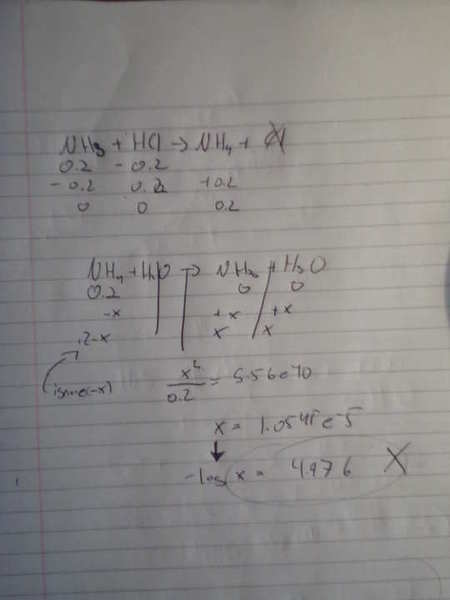# PH/Titration Question

## Homework Statement

Calculate the pH at the equivalence point for the titration of 0.20 M NH3 with 0.20 M HCl? Kb of ammonia is 1.8e-5

This is just a old test I am going over, I managed to get a pH of 4.98. but that is not right the right answer is 5.12 and I cant figure out why. I would assume you could use the henderson equation but I only get 4.98 which is wrong.

Any help?

acid base titration equivalence point calculation

Note: you should not use Henderson-Hasselbalch, however, you should not forget about dilution.

Even if I did not use HH equation I am getting the wrong answer.

Borek
Mentor
So show details of your work, hard to say what you did wrong not knowing what you did.

So show details of your work, hard to say what you did wrong not knowing what you did.
k.... using ICE table below:Borek
Mentor

I did and didn not use the HH eq, not do i understand what you mean by dilution.

I also read link, and this is onlything really useful that I understood but still does not explain what I am doing wrong:
In the case of titration of weak base with strong acid, situation is very similar - pH at the equivalence point is determined by the weak base salt hydrolysis. Thus we need pKa of conjugated acid to calculate H+ and pH. Check lecture and cheat sheet mentioned above for details.

It would be great if you told me what I am doing wrong...

Borek
Mentor
Try here: dilution effects. Generally speaking, you have mixed two solutions, concentrations have changed as the final volume differs from the initial for each reagent.